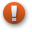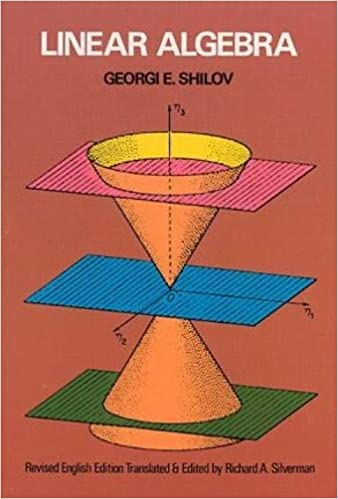# Linear Algebra (Book)This article is a stub. You can help us by editing this page and expanding it.
Information Linear AlgebraGeorgi Shilov English Dover Publications 1 June 1977 400 048663518X 978-0486635187

The textbook Linear Algebra by Georgi Shilov thoroughly covers all major aspects of linear algebra, in addition it covers more geometrically motivated linear algebra in the latter half.

Chapter/Section # Title Page #
Chapter 1: DETERMINANTS
1.1 Number Fields 1
1.2 Problems of the Theory of Systems of Linear Equations 3
1.3 Determinants of Order \(n\) 5
1.4 Properties of Determinants 8
1.5 Cofactors and Minors 12
1.6 Practical Evaluation of Determinants 16
1.7 Cramer's Rule 18
1.8 Minors of Arbitrary Order. Laplace's Theorem 20
1.9 Multiplicative inverses 23
Problems 28
Chapter 2: LINEAR SPACES
2.1 Definitions 31
2.2 Linear Dependence 36
2.3 Bases, Components, Dimension 38
2.4 Subspaces 42
2.5 Linear Manifolds 49
2.6 Hyperplanes 51
2.7 Morphisms of Linear Spaces 53
Problems 56
Chapter 3: SYSTEMS OF LINEAR EQUATIONS
3.1 More on the Rank of a Matrix 58
3.2 Nontrivial Compatibility of a Homogeneous Linear System 60
3.3 The Compatibility Condition for a General Linear System 61
3.4 The General Solution of a Linear System 63
3.4 Geometric Properties of the Solution Space 65
3.4 Methods for Calculating the Rank of a Matrix 67
Problems 71
Chapter 4: LINEAR FUNCTIONS OF A VECTOR ARGUMENT
4.1 Linear Forms 75
4.2 Linear Operators 77
4.3 Sums and Products of Linear Operators 82
4.4 Corresponding Operations on Matrices 84
4.5 Further Properties of Matrix Multiplication 88
4.6 The Range and Null Space of a Linear Operator 93
4.7 Linear Operators Mapping a Space \(K_n\) into Itself 98
4.8 Invariant Subspaces 106
4.9 Eigenvectors and Eigenvalues 108
Problems 113
Chapter 5: COORDINATE TRANSFORMATIONS
5.1 Transformation to a New Basis 118
5.2 Consecutive Transformations 120
5.3 Transformation of the Components of a Vector 121
5.4 Transformation of the Coefficients of a Linear Form 123
5.5 Transformation of the Matrix of a Linear Operator 124
*5.6 Tensors 126
Problems 131
Chapter 6: THE CANONICAL FORM OF THE MATRIX OF A LINEAR OPERATOR
6.1 Canonical Form of the Matrix of a Nilpotent Operator 133
6.2 Algebras. The Algebra of Polynomials 136
6.3 Canonical Form of the Matrix of an Arbitrary Operator 142
6.4 Elementary Divisors 147
6.5 Further Implications 153
6.6 The Real Jordan Canonical Form 155
6.7 Spectra, Jets and Polynomials 160
6.8 Operator Functions and Their Matrices 169
Problems 176
Chapter 7: BILINEAR AND QUADRATIC FORMS
7.1 Bilinear Forms 179
7.3 Reduction of a Quadratic Form to Canonical Form 183
7.4 The Canonical Basis of a Bilinear Form 183
7.5 Construction of a Canonical Basis by Jacobi's Method 183
7.7 Isomorphism of Spaces Equipped with a Bilinear Form 183
*7.8 Multilinear Forms 183
7.9 Bilinear and Quadratic Forms in a Real Space 183
Problems 210
Chapter 8: EUCLIDEAN SPACES
8.1 Introduction 214
8.2 Definition of a Euclidean Space 215
8.3 Basic Metric Concepts 216
8.4 Orthogonal Bases 222
8.5 Perpendiculars 223
8.6 The Orthogonalization Theorem 226
8.7 The Gram Determinant 230
8.8 Incompatible Systems and the Method of Least Squares 234
8.9 Adjoint Operators and Isometry 237
Problems 241
Chapter 9: UNITARY SPACES
9.1 Hermitian Forms 247
9.2 The Scalar Product in a Complex Space 254
9.3 Normal Operators 259
9.4 Applications to Operator Theory in Euclidean Space 263
Problems 271
Chapter 10: QUADRATIC FORMS IN EUCLIDEAN AND UNITARY SPACES
10.1 Basic Theorem on Quadratic Forms in a Euclidean Space 273
10.2 Extremal Properties of a Quadratic Form 276
10.3 Simultaneous Reduction of Two Quadratic Forms 283
10.4 Reduction of the General Equation of a Quadric Surface 287
10.5 Geometric Properties of a Quadric Surface 289
*10.6 Analysis of a Quadric Surface from Its General Equation 300
Problems 310
Chapter 11: FINITE-DIMENSIONAL ALGEBRAS AND THEIR REPRESENTATIONS
11.1 More on Algebras 312
11.2 Representations of Abstract Algebras 313
11.3 Irreducible Representations and Schur's Lemma 314
11.4 Basic Types of Finite-Dimensional Algebras 315
11.5 The Left Regular Representation of a Simple Algebra 318
11.6 Structure of Simple Algebras 320
11.7 Structure of Semisimple Algebras 323
11.8 Representations of Simple and Semisimple Algebras 327
11.9 Some Further Results 331
Problems 332
*Appendix
CATEGORIES OF FINITE-DIMENSIONAL SPACES
A.1 Introduction 335
A.2 The Case of Complete Algebras 338
A.3 The Case of One-Dimensional Algebras 340
A.4 The Case of Simple Algebras 345
A.5 The Case of Complete Algebras of Diagonal Matrices 353
A.6 Categories and Direct Sums 357